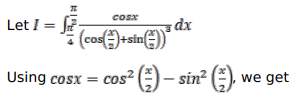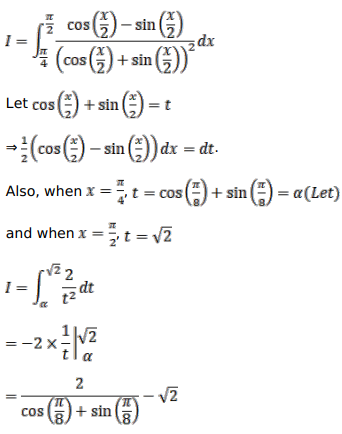# Evaluate the following integralsQuestion:

Evaluate the following integrals

$\int_{\pi / 4}^{\pi / 2} \frac{\cos \theta}{\left.\cos \frac{\theta}{2}+\sin \frac{\theta}{2}\right)^{3}} d \theta$

Solution: